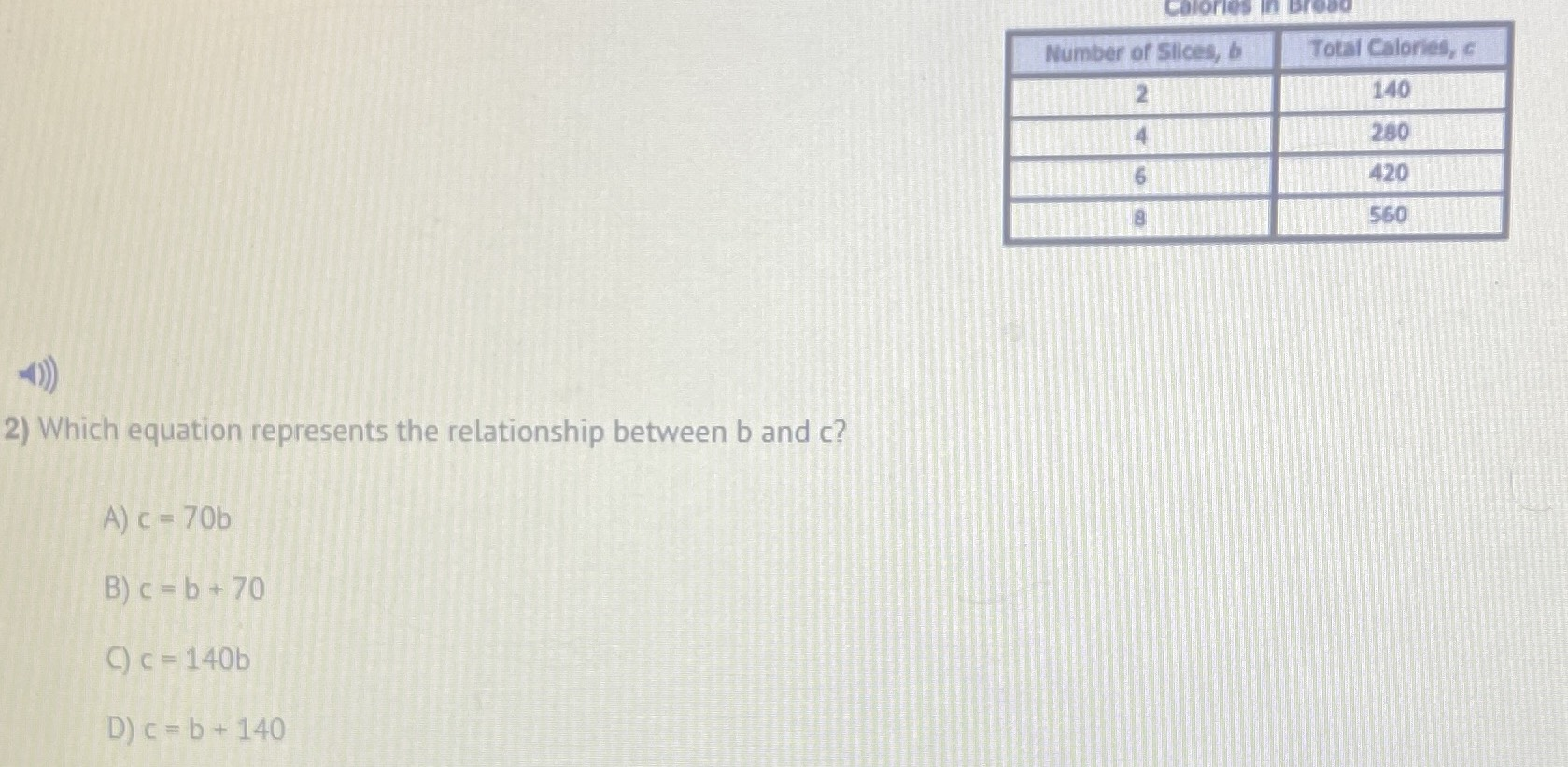### ¿Todavía tienes preguntas de matemáticas?

Pregunte a nuestros tutores expertos
Algebra
Pregunta2) Which equation represents the relationship between $$b$$ and $$c$$ ?

A) $$c = 70 b$$

B) $$c = b + 70$$

C) $$c = 140 b$$

D) $$c = b + 140$$